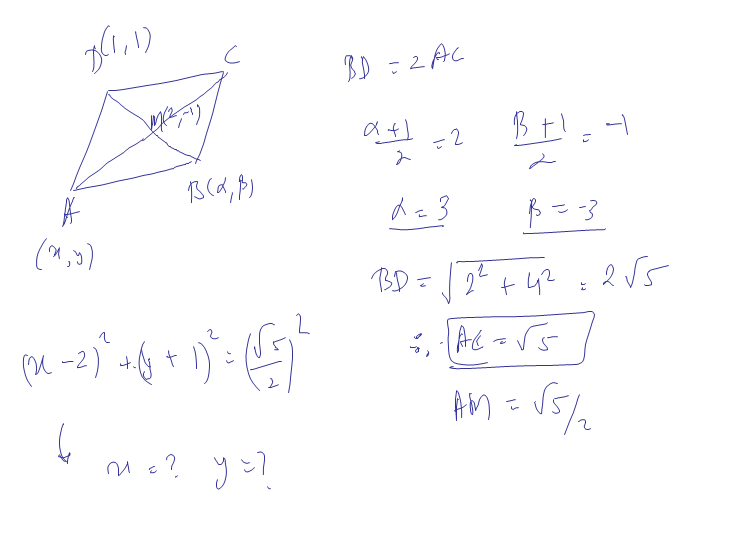# ABCD is a rhombus such that its its diagonals AC and BD intersect at M and satisfy BD=2AC. If the points D and M represent the complex no. 1+i and 2-i respectively, then which complex no. does A represent?Nishant Vora IIT Patna
askIITians Faculty 2467 Points
5 years ago5 years ago
From the given info, DA = ½ DM and is obtained by rotating DM by 900 in anti-clockwise direction. DM = (1+i)-(2-i) = 2i-1.

Hence DA = (2i-1) * i/2 = -1 – i/2.

Hence we get A = (2-i)+(-1-i/2) = 1 – 3i/2